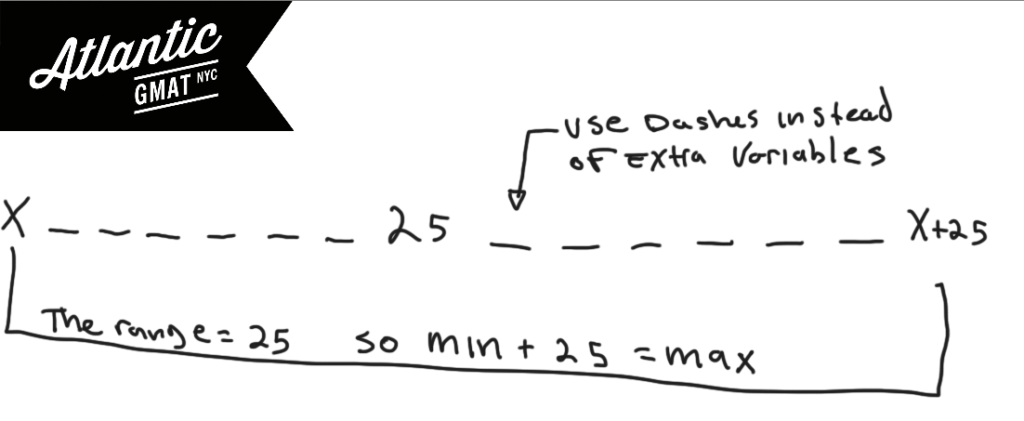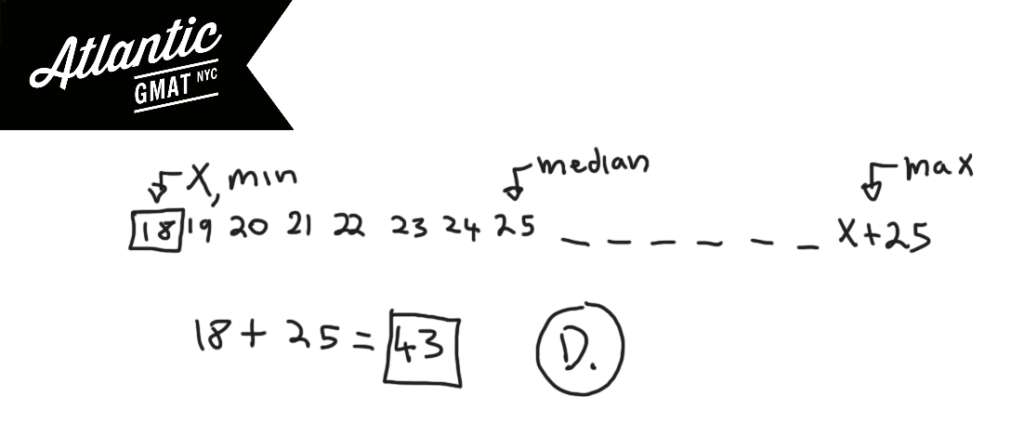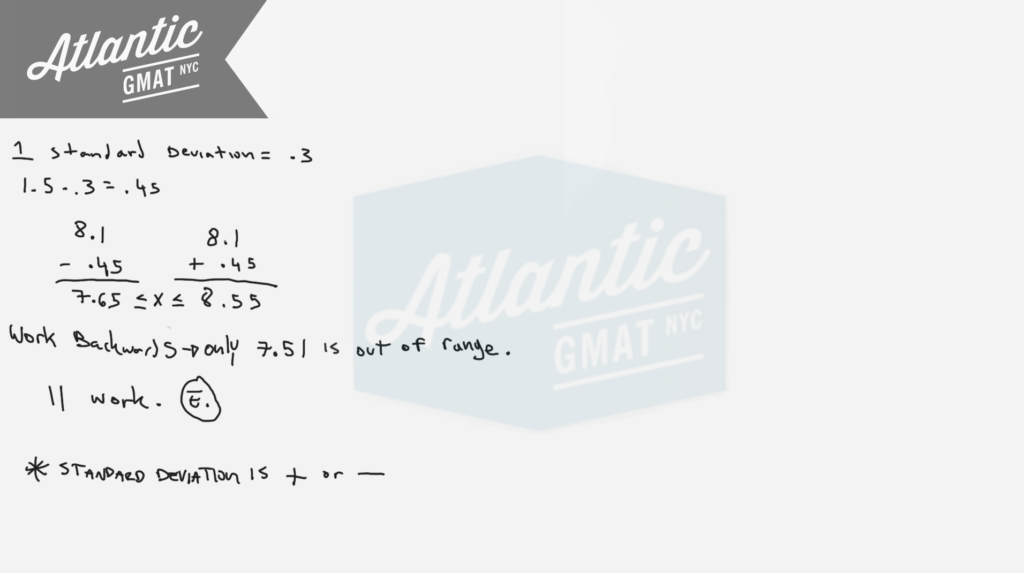Private GMAT Tutoring in NYC & Online

SCORE INTERVAL----------------NUMBER OF SCORES

50-59-------------------------- 2
60-69--------------------------10
70-79--------------------------16
80-89--------------------------27
90-99--------------------------18

The table above shows the distribution of test scores for a group of management trainees. Which score interval contains the median of the 73 scores?

(A) 60-69
(B) 70-79
(C) 80-89
(D) 90-99
(E) It cannot be determined from the information given.

For a certain exam, a score of 58 was 2 standard deviations below mean and a score of 98 was 3 standard deviations above mean. What was the mean score for the exam?

(A) 74
(B) 76
(C) 78
(D) 80
(E) 82

Last month 15 homes were sold in Town X. The average (arithmetic mean) sale price of the homes was \$150,000 and the median sale price was \$130,000. Which of the following statements must be true?

I. At least one of the homes was sold for more than \$165,000.
II. At least one of the homes was sold for more than \$130,0000 and less than \$150,000
III. At least one of the homes was sold for less than \$130,000.

(A) I only
(B) II only
(C) III only
(D) I and II
(E) I and III

A set of 15 different integers has median of 25 and a range of 25. What is greatest possible integer that could be in this set?

(A) 32
(B) 37
(C) 40
(D) 43
(E) 50

This GMAT question falls firmly into statistics. Foggy on median and range? Go back to the GMAT basics! Get this fundamental GMAT content down backwards and forwards so that you can focus on the critical thinking portion of the exam. Median is the middle number of an odd set or the average of the two middle numbers of an even set. Range is the biggest number minus the smallest number (Range = max - min). Let's start by plotting out what we know. Median is spatial so demands a diagram. In a set of 15 numbers the median is the 8th number (7 above it, 7 below it). Especially for statistics set questions avoid creating a million variables for numbers you're not solving for. Let's represent those numbers with dashes and create a variable for the min. Then you can add 25 to that for the max. Keep in mind what we're solving for: the greatest possible integer that could be in this set.We know the range is 25 and that we want to make the max as big as possible. So what should we do with the min? We want to make the min as big as possible so that min + 25 (the max) is as big as possible. You think: let's make the min = median. Good thinking! That would be perfect. But that's not possible as it is a set of 15 different integers. Still, we're on the right track. Let's just go down as little as possible from the median. 24.9999999999. Good thinking again! But, we have to stick with integers. So then you've got 24, 23, 22, 21, 20, 19, 18. Add 25 to 18 and that's the greatest possible integer in the set. Comment with any questions or additions. Happy GMAT studying!7.51 8.22 7.86 8.36
8.09 7.83 8.30 8.01
7.73 8.25 7.96 8.53

A vending machine is designed to dispense 8 ounces of coffee into a cup. After a test that recorded the number of ounces of coffee in each of 1000 cups dispensed by the vending machine, the 12 listed amounts, in ounces, were selected from the data above. If the 1000 recorded amounts have a mean of 8.1 ounces and a standard deviation of 0.3 ounces, how many of the 12 listed amounts are within 1.5 standard deviation of the mean?

(A) Four
(B) Six
(C) Nine
(D) Ten
(E) Eleven

This question is from the the GMAT Prep Test 1 and 2 so if you haven't worked on those yet go ahead and skip this one for now. It's important to remember that standard deviation is plus or minus. So 1 standard deviation out would be 8.4 on the upper end and 7.8 on the lower end. 1.5 standard deviations out is 8.55 on the upper end and 7.65 on the lower end. Now you just have knock out amounts out of that range. Working backwards is faster since it becomes clear that most of the measurements are falling within 1.5 standard deviations of the mean. Let's also remember that we're not basing all of this on the 8 ounces the vending machine was designed to dispense but on the 8.1 mean that was calculated based on the 1000 cups actually dispensed.# CONTACT

Atlantic GMAT

405 East 51st St.

NY, NY 10022

(347) 669-3545

info@AtlanticGMAT.com